Residual Analysis in Linear Regression

Linear regression is a statistical method for for modelling the linear relationship between a dependent variable y (i.e. the one we want to predict) and one or more explanatory or independent variables(X).

This vignette will explain how residual plots generated by the regression function can be used to validate that some of the assumptions that are made about the dataset indicating it is suitable for a linear regression are met.

If you have ever wondered what these mean and how they can help - this is a small guide!

There are a number of assupmtions we made about the data and these must be met for a linear model to work successfully and the standard residual plots can help validate some of these. These are:

• The dataset must have some linear relationship
• Multivariate normality - the dataset variables must be statistically Normally Distributed (i.e. resembling a Bell Curve)
• It must have no or little multicollinearity - this means the independent variables must not be too highly correlated with each other. This can be tested with a Correlation matrix and other tests
• No auto-correlation - Autocorrelation occurs when the residuals are not independent from each other. For instance, this typically occurs in stock prices, where the price is not independent from the previous price.
• Homoscedasticity - meaning that the residuals are equally distributed across the regression line i.e. above and below the regression line and the variance of the residuals should be the same for all predicted scores along the regression line.

Four standard plots can be accessed using the plot() function with the fit variable once the model is generated. These can be used to show if there are problems with the dataset and the model produced that need to be considered in looking at the validity of the model. These are:

• Residuals vs Fitted Plot
• Normal Q–Q (quantile-quantile) Plot
• Scale-Location
• Residuals vs Leverage

The mtcars dataset is used as an example to show the residual plots. The dataset describes the attibutes of various cars and how these relate to the dependent variable mpg i.e. how to things like weight, no of cylinders and no of gears affect miles per gallon (mpg). For this example we will use mpg (mpg) vs weight (wt).

library(ggplot2)
##                    mpg cyl disp  hp drat    wt  qsec vs am gear carb
## Mazda RX4         21.0   6  160 110 3.90 2.620 16.46  0  1    4    4
## Mazda RX4 Wag     21.0   6  160 110 3.90 2.875 17.02  0  1    4    4
## Datsun 710        22.8   4  108  93 3.85 2.320 18.61  1  1    4    1
## Hornet 4 Drive    21.4   6  258 110 3.08 3.215 19.44  1  0    3    1
## Hornet Sportabout 18.7   8  360 175 3.15 3.440 17.02  0  0    3    2
## Valiant           18.1   6  225 105 2.76 3.460 20.22  1  0    3    1

Fitting the Regression Line and its Residuals

Using the mtcars dataset we can use the lm linear regression function to fit a regression line and then plot it to see the results. The plot shows a good looking regression line.

The plot shows graphically the size of the residual value using a colour code (red is longer line to green - smaller line) and size of point. The size of residual is the length of the vertical line from the point to where it meets the regression line.

d <- mtcars
fit <- lm(mpg ~ wt, data = d) # fit the model
d\$predicted <- predict(fit)   # Save the predicted values
d\$residuals <- residuals(fit) # Save the residual values
ggplot(d, aes(x = wt, y = mpg)) +
geom_smooth(method = "lm", se = FALSE, color = "lightgrey") +     # regression line
geom_segment(aes(xend = wt, yend = predicted), alpha = .2) +      # draw line from point to line
geom_point(aes(color = abs(residuals), size = abs(residuals))) +  # size of the points
scale_color_continuous(low = "green", high = "red") +             # colour of the points mapped to residual size - green smaller, red larger
guides(color = FALSE, size = FALSE) +                             # Size legend removed
geom_point(aes(y = predicted), shape = 1) +
theme_bw()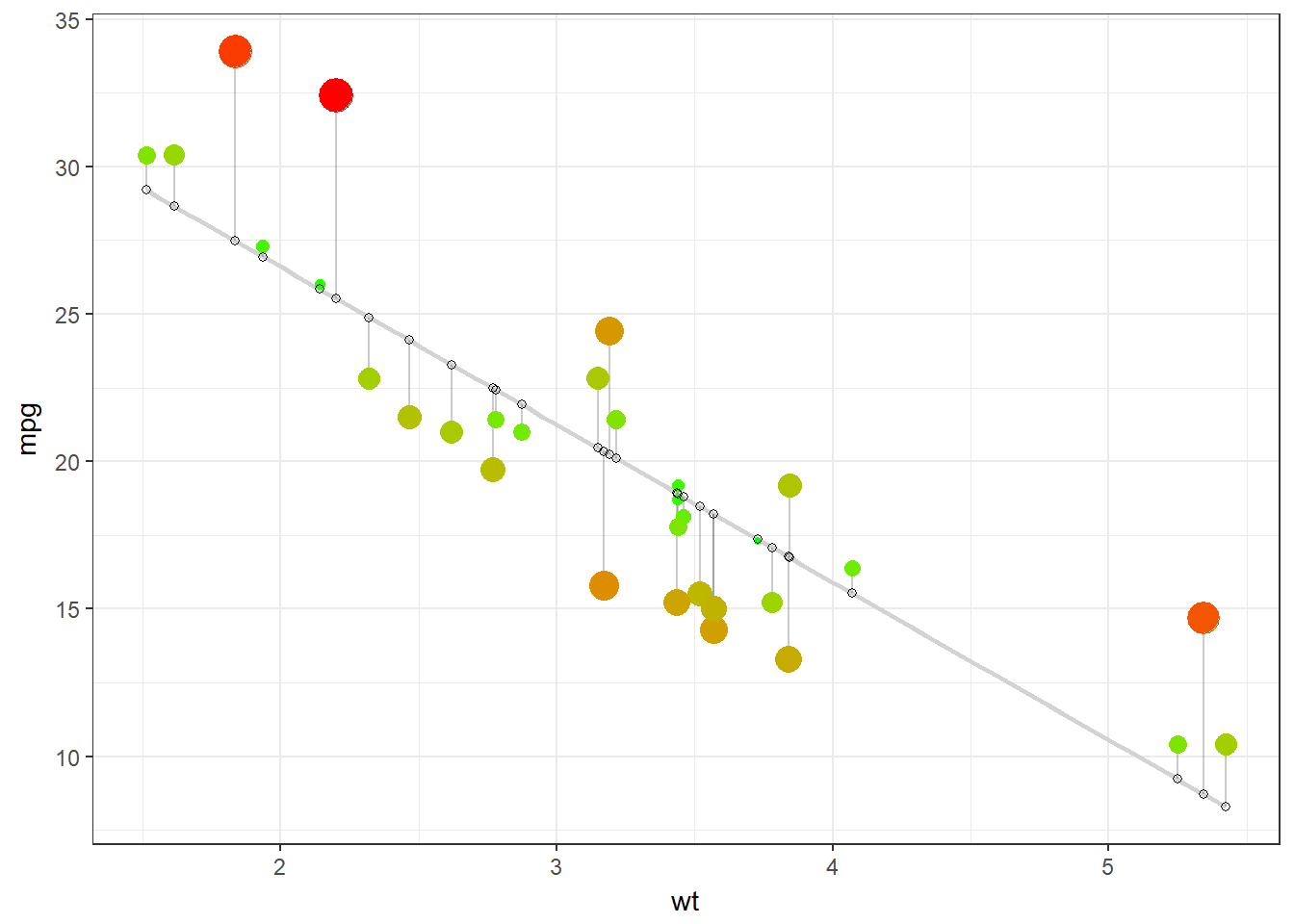summary(fit)
##
## Call:
## lm(formula = mpg ~ wt, data = d)
##
## Residuals:
##     Min      1Q  Median      3Q     Max
## -4.5432 -2.3647 -0.1252  1.4096  6.8727
##
## Coefficients:
##             Estimate Std. Error t value Pr(>|t|)
## (Intercept)  37.2851     1.8776  19.858  < 2e-16 ***
## wt           -5.3445     0.5591  -9.559 1.29e-10 ***
## ---
## Signif. codes:  0 '***' 0.001 '**' 0.01 '*' 0.05 '.' 0.1 ' ' 1
##
## Residual standard error: 3.046 on 30 degrees of freedom
## Multiple R-squared:  0.7528, Adjusted R-squared:  0.7446
## F-statistic: 91.38 on 1 and 30 DF,  p-value: 1.294e-10

Looking at the summary, it has p-value of 1.294e-10, which indicates that there is a highly statistically significant relationship between the two variables. So, why do we need to look at other things like residuals?

P-values by themselves can potentially be misleading without analyis of the residuals to ensure the model does not have any problems.

1. Residuals vs Fitted Plot

As Brian Caffo explains in his book Regression Models for Data Science in R (https://leanpub.com/regmods/read#leanpub-auto-residuals), residuals represent variation left unexplained by the model.

Residual plots are used to look for underlying patterns in the residuals that may mean that the model has a problem.

When using the plot() function, the first plot is the Residuals vs Fitted plot and gives an indication if there are non-linear patterns. For a correct linear regression, the data needs to be linear so this will test if that condition is met.

The example below from Caffo’s book, shows a regression line fit through a number of points.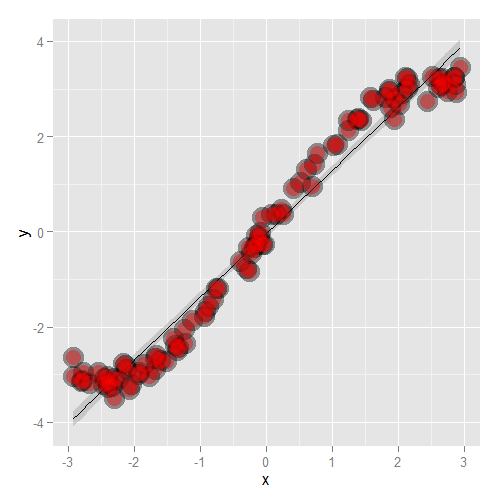However, in the second plot, when we look at the residuals vs X(fitted values) we can see a clear sine curve pattern which indicates that the dataset is not linear. Another model should be used.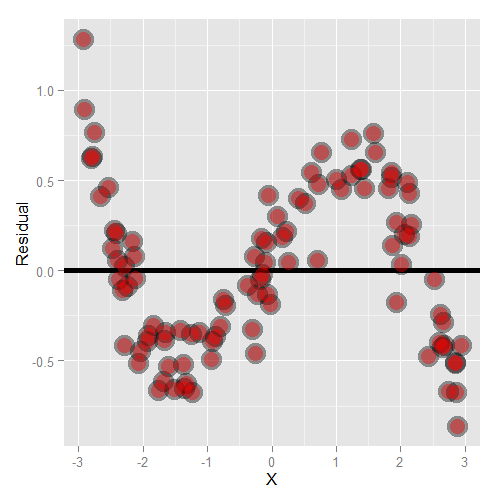For the mtcars data, when we look at the plot below, we see that the data does not have any obvious distinct pattern. While it is slightly curved, it has equally spread residuals around the horizontal line without a distinct pattern.

This is a good indication it is not a non-linear relationship.

plot(fit, which=1, col=c("blue")) # Residuals vs Fitted Plot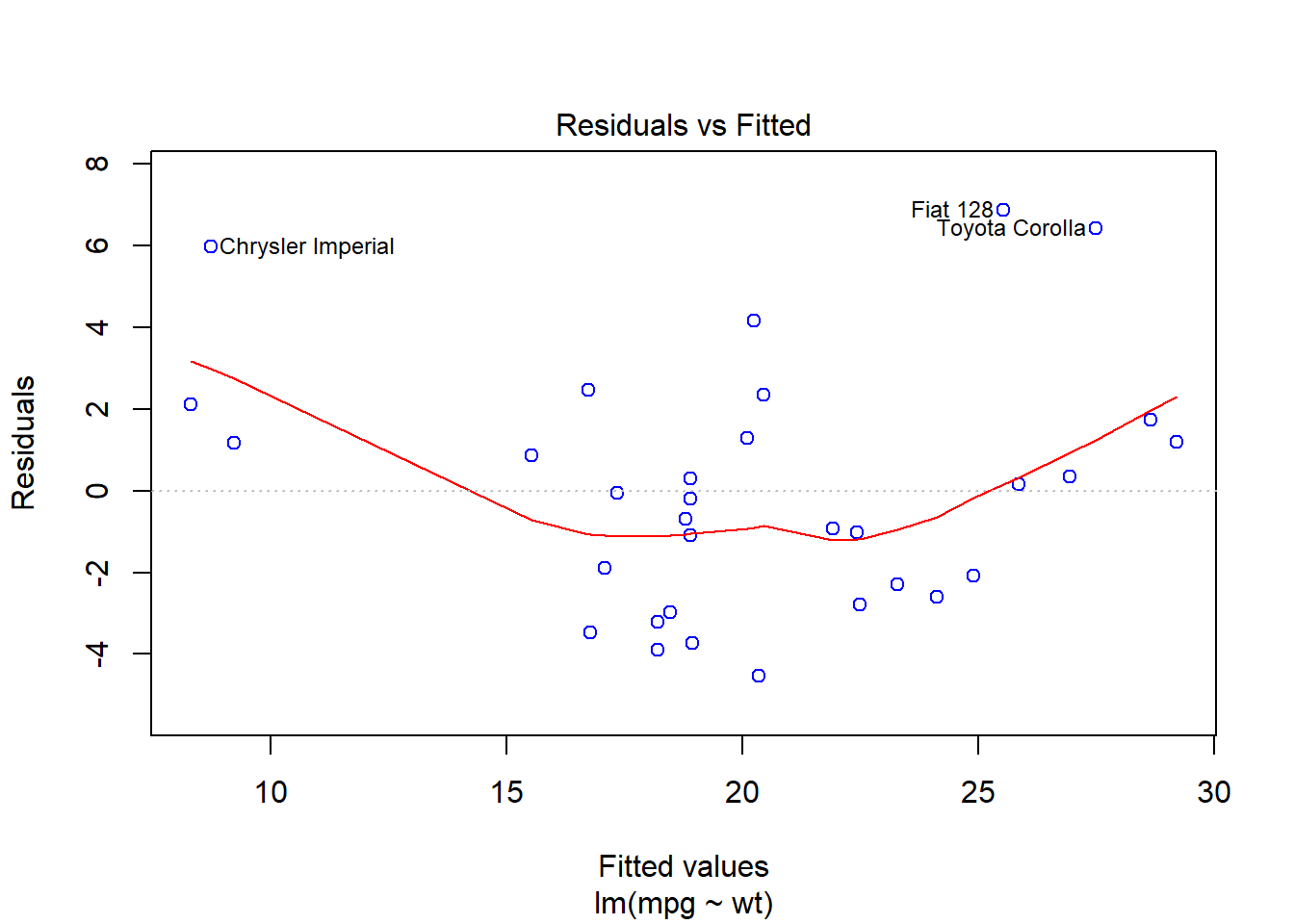2.Normal Q–Q (quantile-quantile) Plot

Residuals should be normally distributed and the Q-Q Plot will show this. If residuals follow close to a straight line on this plot, it is a good indication they are normally distributed.

An example from the University of Virginia(http://data.library.virginia.edu/diagnostic-plots/) shows a good and bad case. Case 1 shows good alighment but Case 2 moves off the line considerable so indicates a problem.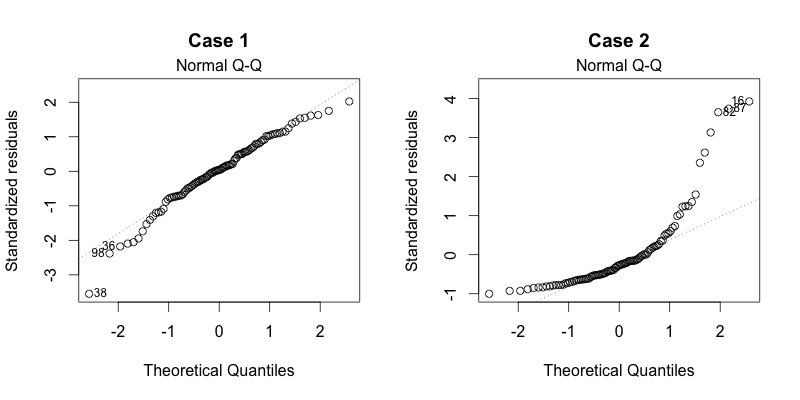For our model, the Q-Q plot shows pretty good alignment to the the line with a few points at the top slightly offset. Probably not significant and a reasonable alignment.

plot(fit, which=2, col=c("red"))  # Q-Q Plot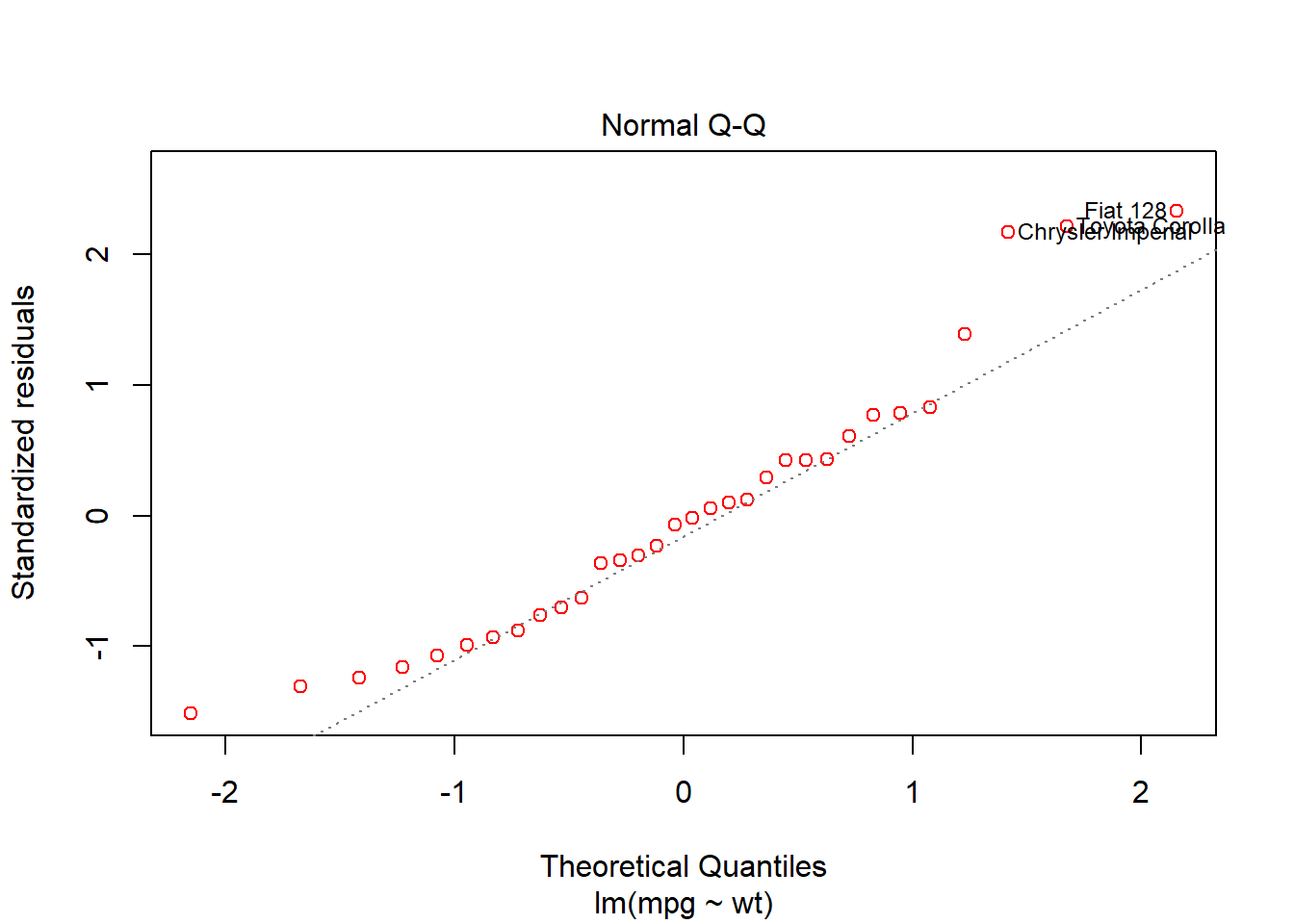3. Scale-Location

This plot test the linear regression assumption of equal variance (homoscedasticity) i.e. that the residuals have equal variance along the regression line. It is also called the Spread-Location plot.

So what does this mean? Here is an example of what it should look like. The residuals have equal variance(occupy equal space) above and below the line and along the length of the line. This is an example from https://stats.stackexchange.com/questions/52089/what-does-having-constant-variance-in-a-linear-regression-model-mean/52107#52107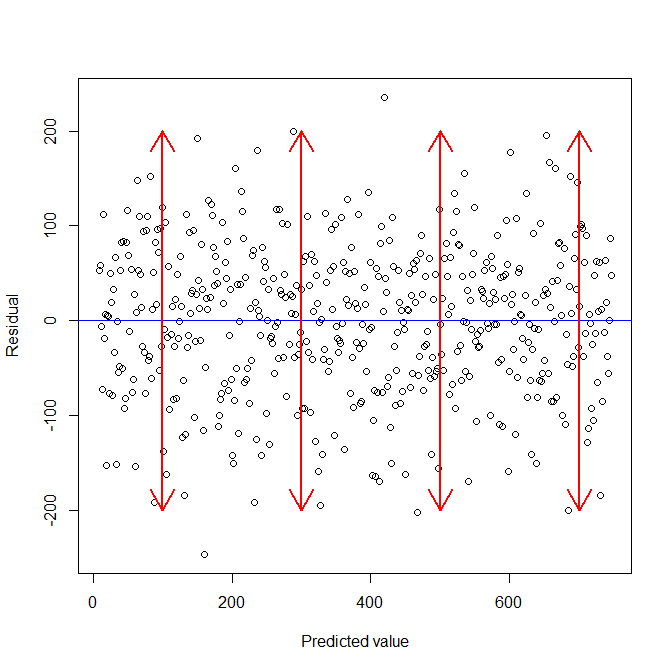Again, an example from the University of Virginia, shows a two Scale-Location plots. Case 1 is more randomly spread above and below and along the line. Case 2 is narrower at the bottom and much wider at the top showing the residuals do not have constant variance and the line is clearnly not horizontal. You can also test using variance calculations for difference parts of the dataset.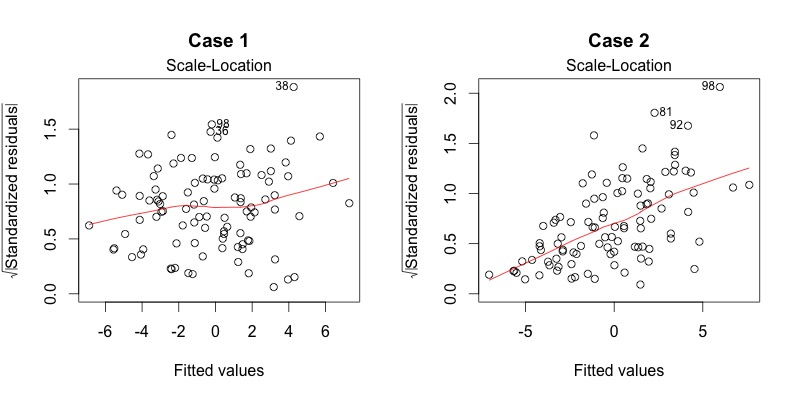For our mtcars example the residuals are reasonably well spread above and below a pretty horizontal line however the beginning of the line does have fewer points so slightly less variance there.

plot(fit, which=3, col=c("blue"))  # Scale-Location Plot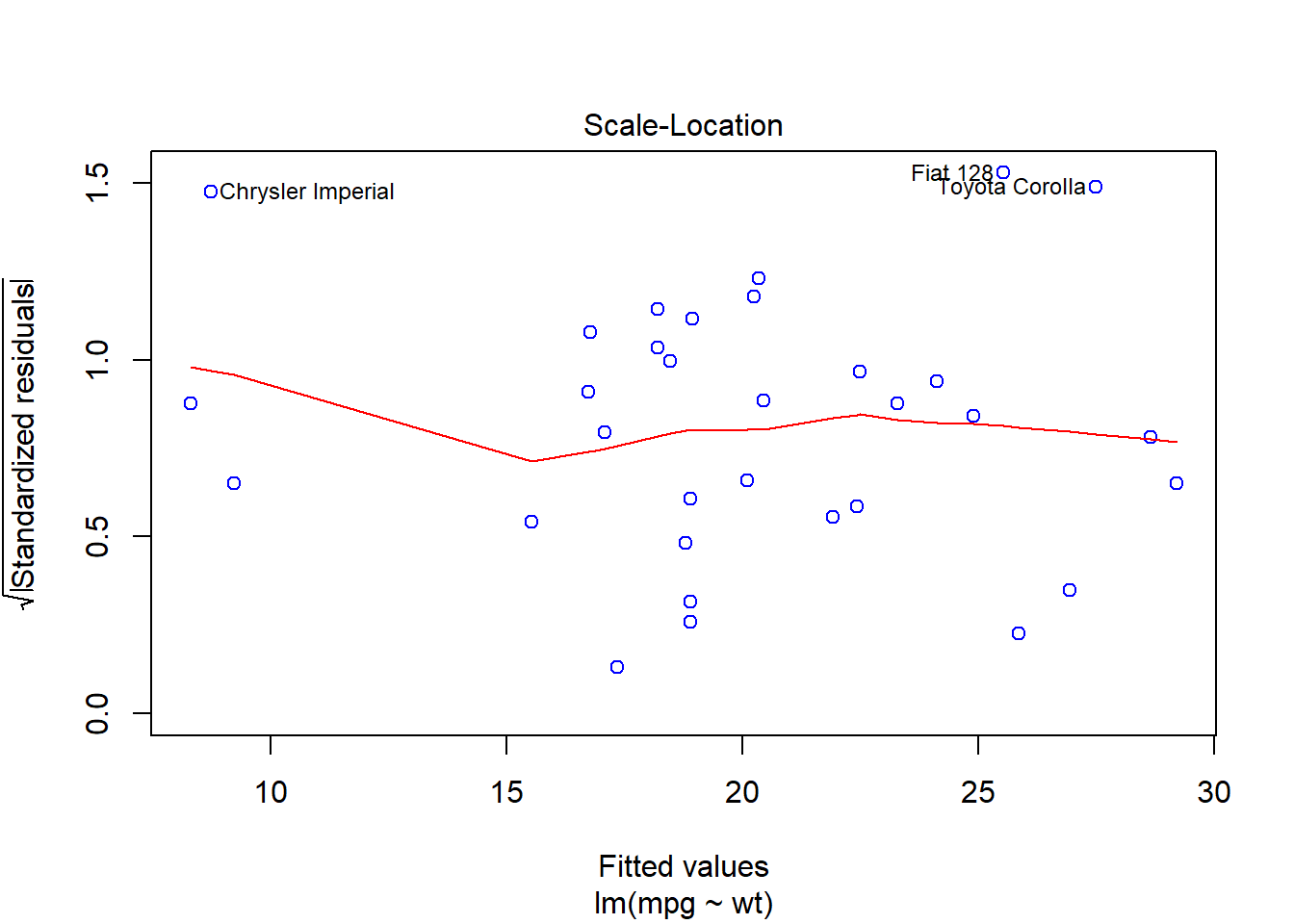4. Residuals vs Leverage

This plot can be used to find influential cases in the dataset. An influential case is one that, if removed, will affect the model so its inclusion or exclusion should be considered.

An influential case may or may not be an outlier and the purpose of this chart is to identify cases that have high influence in the model. Outliers will tend to exert leverage and therefore influence on the model.

An influential case will appear in the top right or bottom left of the chart inside a red line which marks Cook’s Distance. An example is shown below. The case on the right shows one item, the 49th, inside the red dashed line. Removing this from the dataset would have a significant affect on the model, which may or may not be desirable.